# Cost of capital

24 de Apr de 2018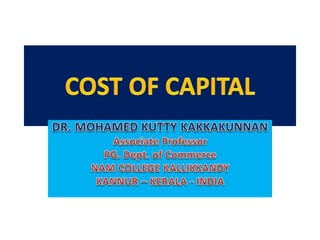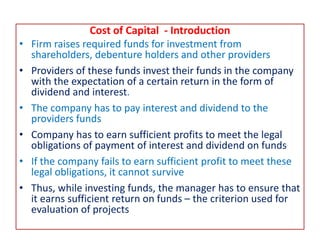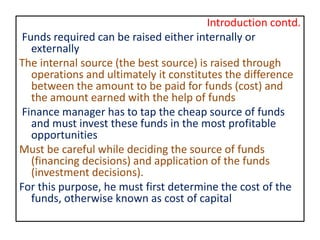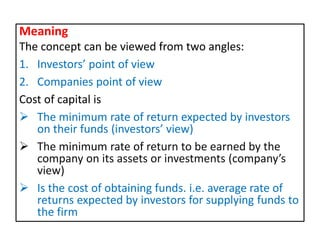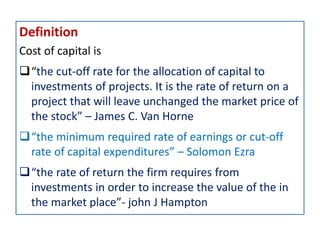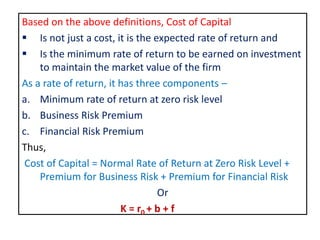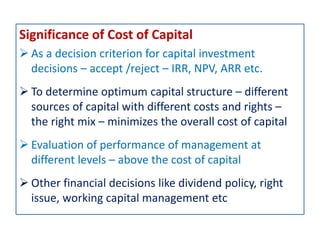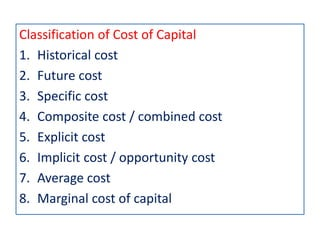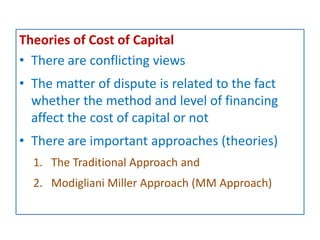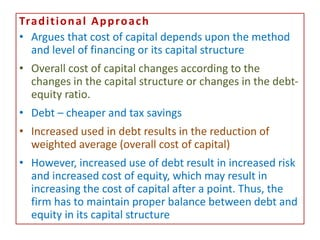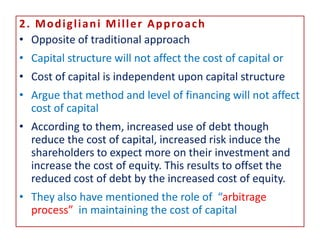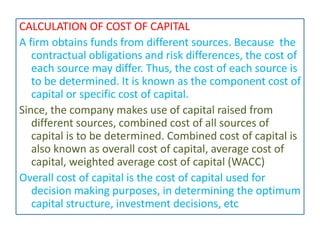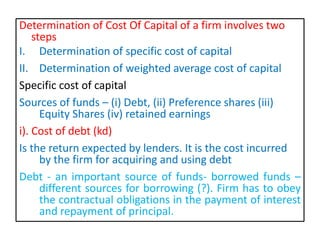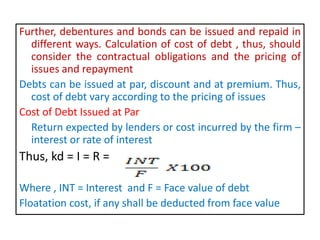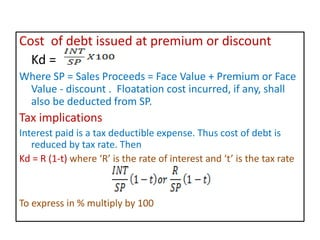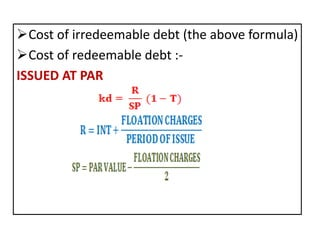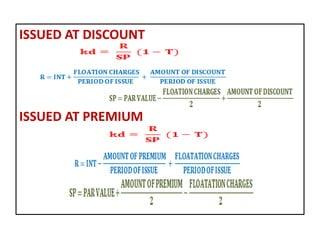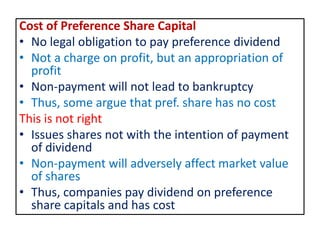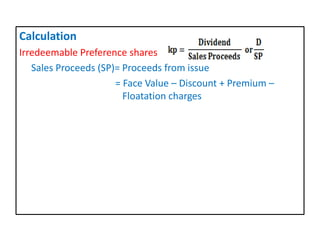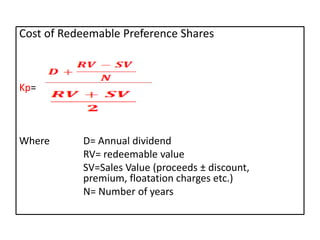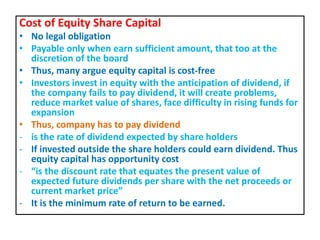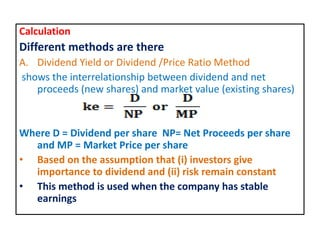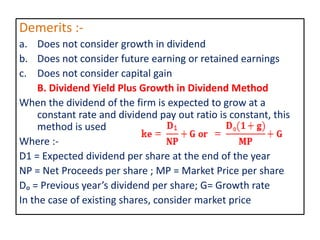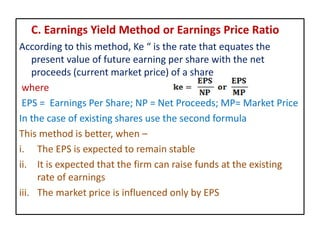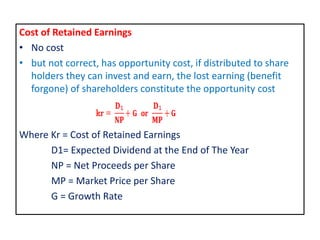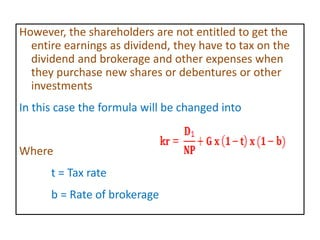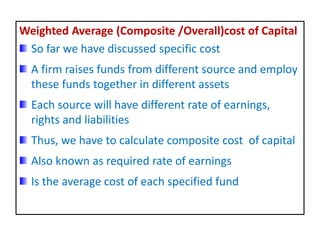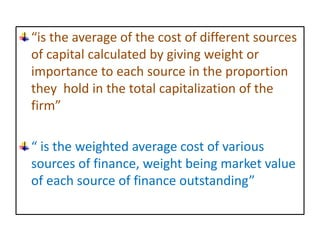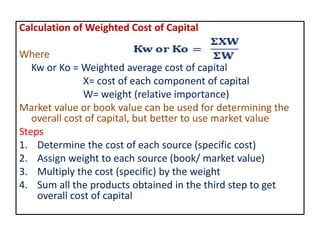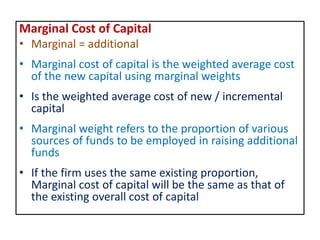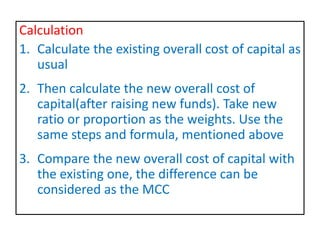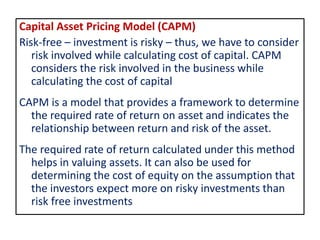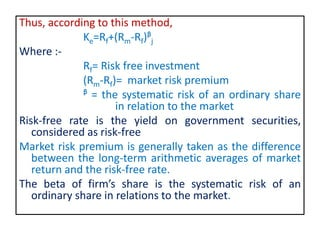1 de 33

### Cost of capital

• 2. Cost of Capital - Introduction • Firm raises required funds for investment from shareholders, debenture holders and other providers • Providers of these funds invest their funds in the company with the expectation of a certain return in the form of dividend and interest. • The company has to pay interest and dividend to the providers funds • Company has to earn sufficient profits to meet the legal obligations of payment of interest and dividend on funds • If the company fails to earn sufficient profit to meet these legal obligations, it cannot survive • Thus, while investing funds, the manager has to ensure that it earns sufficient return on funds – the criterion used for evaluation of projects
• 3. Introduction contd. Funds required can be raised either internally or externally The internal source (the best source) is raised through operations and ultimately it constitutes the difference between the amount to be paid for funds (cost) and the amount earned with the help of funds Finance manager has to tap the cheap source of funds and must invest these funds in the most profitable opportunities Must be careful while deciding the source of funds (financing decisions) and application of the funds (investment decisions). For this purpose, he must first determine the cost of the funds, otherwise known as cost of capital
• 4. Meaning The concept can be viewed from two angles: 1. Investors’ point of view 2. Companies point of view Cost of capital is  The minimum rate of return expected by investors on their funds (investors’ view)  The minimum rate of return to be earned by the company on its assets or investments (company’s view)  Is the cost of obtaining funds. i.e. average rate of returns expected by investors for supplying funds to the firm
• 5. Definition Cost of capital is “the cut-off rate for the allocation of capital to investments of projects. It is the rate of return on a project that will leave unchanged the market price of the stock” – James C. Van Horne “the minimum required rate of earnings or cut-off rate of capital expenditures” – Solomon Ezra “the rate of return the firm requires from investments in order to increase the value of the in the market place”- john J Hampton
• 6. Based on the above definitions, Cost of Capital  Is not just a cost, it is the expected rate of return and  Is the minimum rate of return to be earned on investment to maintain the market value of the firm As a rate of return, it has three components – a. Minimum rate of return at zero risk level b. Business Risk Premium c. Financial Risk Premium Thus, Cost of Capital = Normal Rate of Return at Zero Risk Level + Premium for Business Risk + Premium for Financial Risk Or K = r0 + b + f
• 7. Significance of Cost of Capital  As a decision criterion for capital investment decisions – accept /reject – IRR, NPV, ARR etc.  To determine optimum capital structure – different sources of capital with different costs and rights – the right mix – minimizes the overall cost of capital  Evaluation of performance of management at different levels – above the cost of capital  Other financial decisions like dividend policy, right issue, working capital management etc
• 8. Classification of Cost of Capital 1. Historical cost 2. Future cost 3. Specific cost 4. Composite cost / combined cost 5. Explicit cost 6. Implicit cost / opportunity cost 7. Average cost 8. Marginal cost of capital
• 9. Theories of Cost of Capital • There are conflicting views • The matter of dispute is related to the fact whether the method and level of financing affect the cost of capital or not • There are important approaches (theories) 1. The Traditional Approach and 2. Modigliani Miller Approach (MM Approach)
• 10. Traditional Approach • Argues that cost of capital depends upon the method and level of financing or its capital structure • Overall cost of capital changes according to the changes in the capital structure or changes in the debt- equity ratio. • Debt – cheaper and tax savings • Increased used in debt results in the reduction of weighted average (overall cost of capital) • However, increased use of debt result in increased risk and increased cost of equity, which may result in increasing the cost of capital after a point. Thus, the firm has to maintain proper balance between debt and equity in its capital structure
• 11. 2. Modigliani Miller Approach • Opposite of traditional approach • Capital structure will not affect the cost of capital or • Cost of capital is independent upon capital structure • Argue that method and level of financing will not affect cost of capital • According to them, increased use of debt though reduce the cost of capital, increased risk induce the shareholders to expect more on their investment and increase the cost of equity. This results to offset the reduced cost of debt by the increased cost of equity. • They also have mentioned the role of “arbitrage process” in maintaining the cost of capital
• 12. CALCULATION OF COST OF CAPITAL A firm obtains funds from different sources. Because the contractual obligations and risk differences, the cost of each source may differ. Thus, the cost of each source is to be determined. It is known as the component cost of capital or specific cost of capital. Since, the company makes use of capital raised from different sources, combined cost of all sources of capital is to be determined. Combined cost of capital is also known as overall cost of capital, average cost of capital, weighted average cost of capital (WACC) Overall cost of capital is the cost of capital used for decision making purposes, in determining the optimum capital structure, investment decisions, etc
• 13. Determination of Cost Of Capital of a firm involves two steps I. Determination of specific cost of capital II. Determination of weighted average cost of capital Specific cost of capital Sources of funds – (i) Debt, (ii) Preference shares (iii) Equity Shares (iv) retained earnings i). Cost of debt (kd) Is the return expected by lenders. It is the cost incurred by the firm for acquiring and using debt Debt - an important source of funds- borrowed funds – different sources for borrowing (?). Firm has to obey the contractual obligations in the payment of interest and repayment of principal.
• 14. Further, debentures and bonds can be issued and repaid in different ways. Calculation of cost of debt , thus, should consider the contractual obligations and the pricing of issues and repayment Debts can be issued at par, discount and at premium. Thus, cost of debt vary according to the pricing of issues Cost of Debt Issued at Par Return expected by lenders or cost incurred by the firm – interest or rate of interest Thus, kd = I = R = Where , INT = Interest and F = Face value of debt Floatation cost, if any shall be deducted from face value
• 15. Cost of debt issued at premium or discount Kd = Where SP = Sales Proceeds = Face Value + Premium or Face Value - discount . Floatation cost incurred, if any, shall also be deducted from SP. Tax implications Interest paid is a tax deductible expense. Thus cost of debt is reduced by tax rate. Then Kd = R (1-t) where ‘R’ is the rate of interest and ‘t’ is the tax rate To express in % multiply by 100
• 16. Cost of irredeemable debt (the above formula) Cost of redeemable debt :- ISSUED AT PAR
• 18. Cost of Preference Share Capital • No legal obligation to pay preference dividend • Not a charge on profit, but an appropriation of profit • Non-payment will not lead to bankruptcy • Thus, some argue that pref. share has no cost This is not right • Issues shares not with the intention of payment of dividend • Non-payment will adversely affect market value of shares • Thus, companies pay dividend on preference share capitals and has cost
• 19. Calculation Irredeemable Preference shares Sales Proceeds (SP)= Proceeds from issue = Face Value – Discount + Premium – Floatation charges
• 20. Cost of Redeemable Preference Shares Kp= Where D= Annual dividend RV= redeemable value SV=Sales Value (proceeds ± discount, premium, floatation charges etc.) N= Number of years
• 21. Cost of Equity Share Capital • No legal obligation • Payable only when earn sufficient amount, that too at the discretion of the board • Thus, many argue equity capital is cost-free • Investors invest in equity with the anticipation of dividend, if the company fails to pay dividend, it will create problems, reduce market value of shares, face difficulty in rising funds for expansion • Thus, company has to pay dividend - is the rate of dividend expected by share holders - If invested outside the share holders could earn dividend. Thus equity capital has opportunity cost - “is the discount rate that equates the present value of expected future dividends per share with the net proceeds or current market price” - It is the minimum rate of return to be earned.
• 22. Calculation Different methods are there A. Dividend Yield or Dividend /Price Ratio Method shows the interrelationship between dividend and net proceeds (new shares) and market value (existing shares) Where D = Dividend per share NP= Net Proceeds per share and MP = Market Price per share • Based on the assumption that (i) investors give importance to dividend and (ii) risk remain constant • This method is used when the company has stable earnings
• 23. Demerits :- a. Does not consider growth in dividend b. Does not consider future earning or retained earnings c. Does not consider capital gain B. Dividend Yield Plus Growth in Dividend Method When the dividend of the firm is expected to grow at a constant rate and dividend pay out ratio is constant, this method is used Where :- D1 = Expected dividend per share at the end of the year NP = Net Proceeds per share ; MP = Market Price per share Dₒ = Previous year’s dividend per share; G= Growth rate In the case of existing shares, consider market price
• 24. C. Earnings Yield Method or Earnings Price Ratio According to this method, Ke “ is the rate that equates the present value of future earning per share with the net proceeds (current market price) of a share where EPS = Earnings Per Share; NP = Net Proceeds; MP= Market Price In the case of existing shares use the second formula This method is better, when – i. The EPS is expected to remain stable ii. It is expected that the firm can raise funds at the existing rate of earnings iii. The market price is influenced only by EPS
• 25. Cost of Retained Earnings • No cost • but not correct, has opportunity cost, if distributed to share holders they can invest and earn, the lost earning (benefit forgone) of shareholders constitute the opportunity cost Where Kr = Cost of Retained Earnings D1= Expected Dividend at the End of The Year NP = Net Proceeds per Share MP = Market Price per Share G = Growth Rate
• 26. However, the shareholders are not entitled to get the entire earnings as dividend, they have to tax on the dividend and brokerage and other expenses when they purchase new shares or debentures or other investments In this case the formula will be changed into Where t = Tax rate b = Rate of brokerage
• 27. Weighted Average (Composite /Overall)cost of Capital So far we have discussed specific cost A firm raises funds from different source and employ these funds together in different assets Each source will have different rate of earnings, rights and liabilities Thus, we have to calculate composite cost of capital Also known as required rate of earnings Is the average cost of each specified fund
• 28. “is the average of the cost of different sources of capital calculated by giving weight or importance to each source in the proportion they hold in the total capitalization of the firm” “ is the weighted average cost of various sources of finance, weight being market value of each source of finance outstanding”
• 29. Calculation of Weighted Cost of Capital Where Kw or Ko = Weighted average cost of capital X= cost of each component of capital W= weight (relative importance) Market value or book value can be used for determining the overall cost of capital, but better to use market value Steps 1. Determine the cost of each source (specific cost) 2. Assign weight to each source (book/ market value) 3. Multiply the cost (specific) by the weight 4. Sum all the products obtained in the third step to get overall cost of capital
• 30. Marginal Cost of Capital • Marginal = additional • Marginal cost of capital is the weighted average cost of the new capital using marginal weights • Is the weighted average cost of new / incremental capital • Marginal weight refers to the proportion of various sources of funds to be employed in raising additional funds • If the firm uses the same existing proportion, Marginal cost of capital will be the same as that of the existing overall cost of capital
• 31. Calculation 1. Calculate the existing overall cost of capital as usual 2. Then calculate the new overall cost of capital(after raising new funds). Take new ratio or proportion as the weights. Use the same steps and formula, mentioned above 3. Compare the new overall cost of capital with the existing one, the difference can be considered as the MCC
• 32. Capital Asset Pricing Model (CAPM) Risk-free – investment is risky – thus, we have to consider risk involved while calculating cost of capital. CAPM considers the risk involved in the business while calculating the cost of capital CAPM is a model that provides a framework to determine the required rate of return on asset and indicates the relationship between return and risk of the asset. The required rate of return calculated under this method helps in valuing assets. It can also be used for determining the cost of equity on the assumption that the investors expect more on risky investments than risk free investments
• 33. Thus, according to this method, Ke=Rf+(Rm-Rf)ᵝj Where :- Rf= Risk free investment (Rm-Rf)= market risk premium ᵝ = the systematic risk of an ordinary share in relation to the market Risk-free rate is the yield on government securities, considered as risk-free Market risk premium is generally taken as the difference between the long-term arithmetic averages of market return and the risk-free rate. The beta of firm’s share is the systematic risk of an ordinary share in relations to the market.